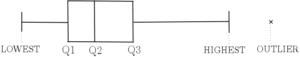# Box Plots

Box plots (or box and whisker plots) provide a convenient way to look at the distribution of a dataset by first identifying the quartiles. Outliers are those values which do not seem to fit with the rest of the data very well. This page will show you how to identify outliers as well as construct box plots and use them in statistical analysis. Note that you may be asked to do this for a given dataset but you may also be asked to do it for a dataset that you are already familiar with. See more on this.

## Box Plots

In order to draw a box plot for a given set of data, one must know the lowest value, the lower quartile (Q1), the median (Q2), the upper quartile (Q3) and the largest value. See more on measures of central tendency and measures of variation. In addition, the possibility of there being outliers and anomalous data entries must be considered. Outliers are determined by a given rule and are usually identified on a box plot by a cross. See more on outliers below. As opposed to a cumulative frequency diagram, values on a box plot are identified horizontally:The gap between the lowest value and Q1 is joined by a horizontal line. This is also true for the gap between Q3 and the highest value. The gaps between Q1/Q2 and Q2/Q3 are shown with boxes. Each of the gaps represent intervals that contain 25% of the data excluding any outliers. This allows the reader to see the ‘spread’ of the data (see ‘Measures of Variation‘). See Example 1 for an example of how to construct a box plot and Example 2 for a demonstration of how to ‘clean’ the data of anomalies and identify outliers before constructing the box plot for a given dataset.  Note that some of the significant data points coincide – also see Example 2 for this.

## Outliers

Outliers are values in a dataset that appear to be suspicious in that they don’t seem to fit with the other data values. Some outliers are valid and should be kept as they legitimately affect the measures of central tendency and variation. Others, however, should be removed before any analysis is performed as they are present, for whatever reason, due to error. These outliers are known as ‘anomalies‘. The process of removing anomalies is known as ‘cleaning the data’

In order to find an outlier (anomaly or not), a rule for identifying outliers must be specified. These are commonly given as follows:

• An outlier may be identified as any value less than ${\text{Q1}}-m\times{\text{IQR}}$ or more than ${\text{Q3}}+m\times {\text{IQR}}$ for some specified value m….
• …or an outlier may be identified as the ${\text{mean}}\pm n\times {\text{standard deviation}}$ for some specified value n.

See more on the quartiles and the interquartile range (IQR) or click here for more on mean and standard deviation. See Example 2 below for a demonstration of identifying outliers and cleaning data before constructing a box plot.

## Box Plot Examples

This dataset shows the ages of 15 female gorillas kept at a conservation park in the Congo:

8, 30, 5, 14, 61, 15, 52, 25, 22, 32, 43, 7, 41, 18, 55

1. Identify the outliers, if any, for this dataset using the following rule for outliers:

either less than ${\text{Q1}}-1.5\times{\text{IQR}}$ or more than ${\text{Q3}}+1.5\times {\text{IQR}}$

2. Construct a box plot to display the ages of the female gorillas.

The statistics of the male gorillas at the same conservation park are calculated as follows:

Lowest=2, Q1=10, Median=22, Q3=34, Highest=53

3. Compare the ages of the female gorillas with the ages of the male gorillas.

Click here for more statistical analysis using this example (Ranges – Example 1).

The following dataset shows the weights of 8 newborn babies recorded by a labour unit in one hour at a given hospital:

2.6kg, 58kg, 3.3kg, 2.8kg, 0.7kg, 4.1kg, 3.5kg, 3.9kg

1. Clean the dataset and identify any outliers that occur greater than two standard deviations away from the mean.
2. Show the clean dataset in a box plot.

…coming soon.